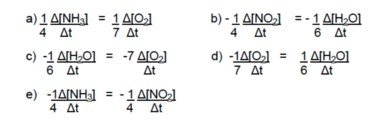# Problem: Using the format given in your text, which of the following rate equalities is correct for the following reaction? 4 NH3(g) + 7 O2 (g) → 4 NO2(g) + 6 H2O(g)

###### Problem Details

Using the format given in your text, which of the following rate equalities is correct for the following reaction?

4 NH3(g) + 7 O2 (g) → 4 NO2(g) + 6 H2O(g)